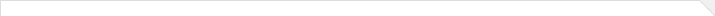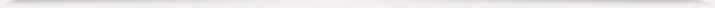`The theory of tetragonal curves is first applied to the study of discrete integrable systems. Based on the discrete Lenard equation, we derive a hierarchy of Blaszak-Marciniak 4-field lattice equation associated with the 4×4 matrix spectral problem. Resorting to the characteristic polynomial of the Lax matrix for the hierarchy of Blaszak-Marciniak 4-field lattice equation, we introduce a tetragonal curve, a Baker-Akhiezer function, and three meromorphic functions on it. We study algebro-geometric properties of the tetragonal curve and asymptotic behaviors of the Baker-Akhiezer function and meromorphic functions near two infinite points. The straightening out of various flows is exactly given by means of the Abel map and the meromorphic differential. We finally obtain Riemann theta function solutions of the entire Blaszak-Marciniak 4-field lattice hierarchy.`海报